In the following $$\mathbf{X}\in\mathbb{R}^{n\times p}$$ is a matrix of $$n$$ rows and $$p$$ columns describing the covariate part of a data set in a mono-block context. If this is a multi-block analysis, with one block for Proteomics, one block for DNA methylation for example and other $$K-2$$ blocks, then the covariate data set is built on $$K$$ blocks and is denoted as $\mathbf{X}_s=\{\mathbf{X}_{proteo},\mathbf{X}_{DNA},\cdots\}\in \mathbb{R}^{n\times p_{proteo}}\times\mathbb{R}^{n\times p_{DNA}}\times\cdots$ $$\mathbf{X}_s$$ is treated with list() structure by the package, so in case of multi-block analysis, thanks to present your dataset such as

# For no named matrices
Xs <- list(X1,X2,X3)

# For names matrices
Xs <- list(X_dna=X1,X_proteo=X2,X_RNA=X3)


if the covariate part is filled with only one data set, it is possible to use the list() structure or not.

In the other cases, the number of variables in each matrix can be different. In the previous examples we have denoted by $$p_{proteo}$$ and $$p_{DNA}$$ two of the different number of variables. It is knowd that Proteomics data-sets are filled with hundreds of variables while DNA methylation data sets gather hundreds of thousands of variables.

The supervizion part is described by the $$\mathbf{Y}\in\mathbb{R}^{n\times q}$$ matrix. In case of regression it can be a vector for univariate case ($$q=1$$) or a matrix or even a data.frame. In multi-variate regression, $$q>1$$, it can be a matrix or a data.frame. In case on classification, $$\mathbf{Y}$$ must be unidimensionnal but it can be a vector or a matrix or even a data.frame. The argument mode permits to choose between regression mode, then use reg, and classification mode, then use something else.

In the $$\mathbf{X}_s$$ and $$\mathbf{Y}$$ data sets, the same numbers of individuals are described, $$n$$ and each of them are on the same row

No individual can be missing in all the $$\mathbf{X}$$ matrices and no missing entry is allowed in the $$\mathbf{Y}$$ dataset.

# Introduction

The present package [based on @lorenzo2019supervised]. It permits to take into account missing values in a multi-block context for supervized problems. It also permits to deal with data analyses with no missing values. Regression or classification regression paradigms are accepted.

Only two parameters must to be fixed by the user :

• R which is the number of components, analogous to the number of components in PCA.

• $$L_0\in\mathbb{N}^\star$$ (or $$\lambda\in[0,1]$$) which is the regularization coefficient. $$L_0$$ can be directly interpreted as the maximum number of variables to be selected in the model.and $$\lambda$$ is the minimum of correlation value under which an interaction between a variable of a block $$\mathbf{X}$$ and one of the $$\mathbf{Y}$$ variables is not taken into account.

Those two parameters are to be fixed with cross validation. We have developped a parallelizable code, using doParallel package [see @doparalelManual]. This is transparent for the user, which hase just to fix the number of cpus to be used. The corresponding parameter is denoted as NCORES. If it equals $$1$$, then no parallelization structure is deployed.

library(ddsPLS)


In the following, only regression examples are shown. To perform classification analyses, please change the argument

mode


to lda of logit if you want to perform classification thanks to linear discriminant analysis or logistic regression (only 2 classes allowed for logit).

## Classification case

data("penicilliumYES")
X <- penicilliumYES$X X <- scale(X[,which(apply(X,2,sd)>0)]) Xs <- list(X[,1:1000],X[,-(1:1000)]) Xs[][1:5,]=Xs[][6:10,] <- NA Y <- as.factor(unlist(lapply(c("Melanoconidiu","Polonicum","Venetum"),function(tt){rep(tt,12)}))) mddsPLS_model_class <- mddsPLS(Xs = Xs,Y = Y,L0=3,R = 2,mode = "lda")  is considered a mult-block classification case analysis with missing samples. mode=“lda” is used since there are 3 classes to discriminate. # Mono-block The here used method can be used on mono and multi-block datasets with no missing values. In the case on mono-block datasets, there is no current developpment to deal with missing samples. Actually this would imply that for a given individual, no covariate is known. Which block any inference. ## Regression case {.tabset} The regression case has been treated through a toy example well know dataset in that section. ### Build a model {.tabset} We have worked on the Liver Toxicity dataset [see @bushel2007simultaneous]. This data set contains the expression measure of 3116 genes and 10 clinical measurements for 64 subjects (rats) that were exposed to non-toxic, moderately toxic or severely toxic doses of acetaminophen in a controlled experiment. Therefore the structure is : $\mathbf{X}\in\mathbb{R}^{64\times3116},\mathbf{Y}\in\mathbb{R}^{64\times10}$ The following commands permit to create the model. data("liverToxicity") X <- scale(liverToxicity$gene)
Y <- scale(liverToxicity$clinic) mddsPLS_model_reg <- mddsPLS(Xs = X,Y = Y,L0=10,R = 3, mode = "reg")  #### Plot Method {.tabset} The Plot mehtod permits many types of representation. Among which • weights which shows all the weights under barplot representations. If super is set to TRUE, then the super-weights are represented. In the mono-block case, super-weights are equal to weights. • heatmap which shows the selected variables (for $$\mathbf{X}$$ and $$\mathbf{Y}$$) for the all individuals. The following sections detailed the discussed method though those parametizations. ##### Super-Weight representation The following figure permits to represent the super-weights for the first and the second components for block 1 (mono-block case actually) and block Y. mar_left parameter adds extra values on the left of the plot, usefull to see variable names. plot(mddsPLS_model_reg,vizu = "weights",variance = "Linear", super = T,comp = c(1,2),addY = T,mar_left = 3,mar_bottom = 3, pos_legend = "topright",reorder_Y = T,legend.cex = 1.5)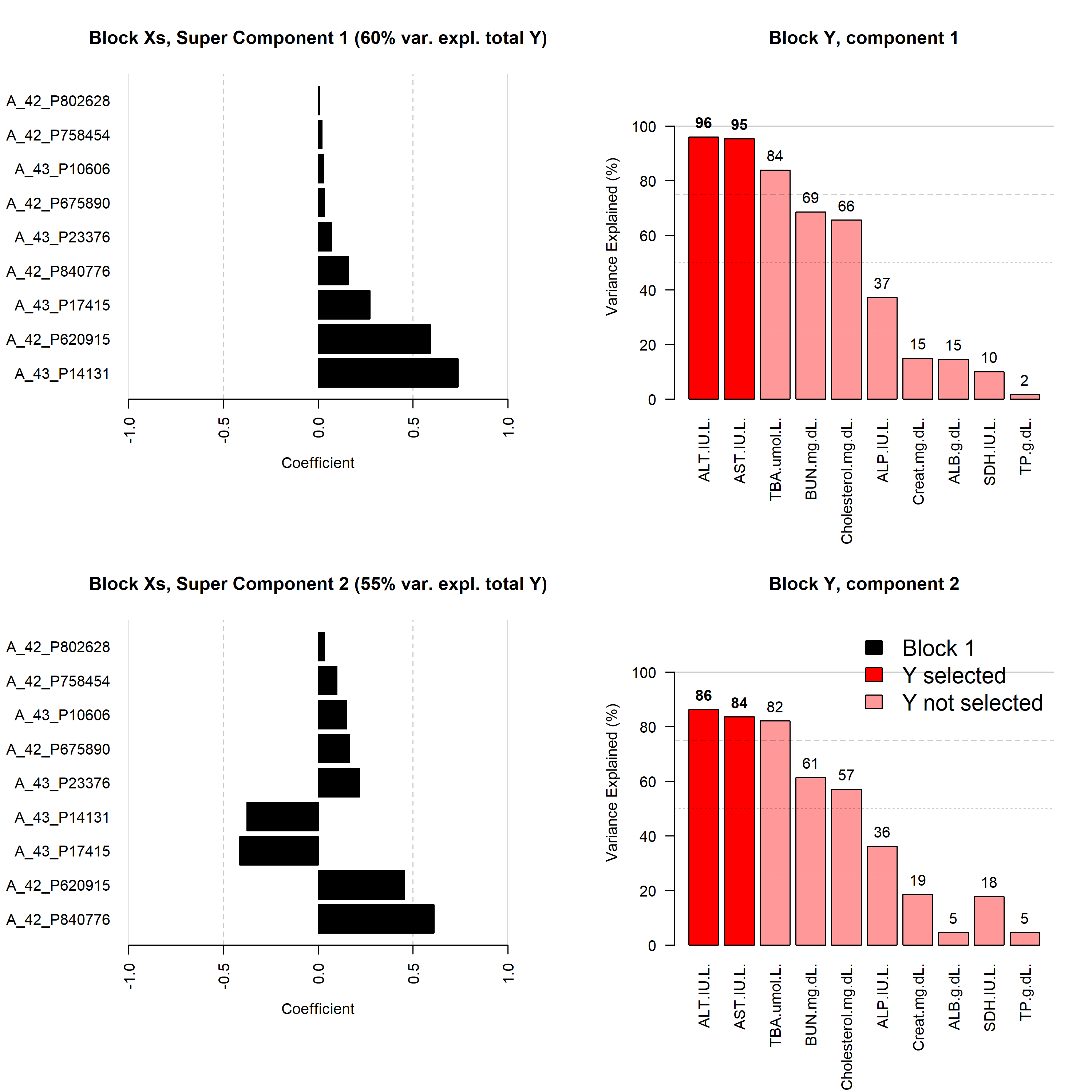##### Heatmap representation Heatmap permit to appreciate the discriminative aspect of variables according to their individual representations. It has been decided to plot heatmaps component per component, only the first one here plot(mddsPLS_model_reg,vizu = "heatmap",comp = 1)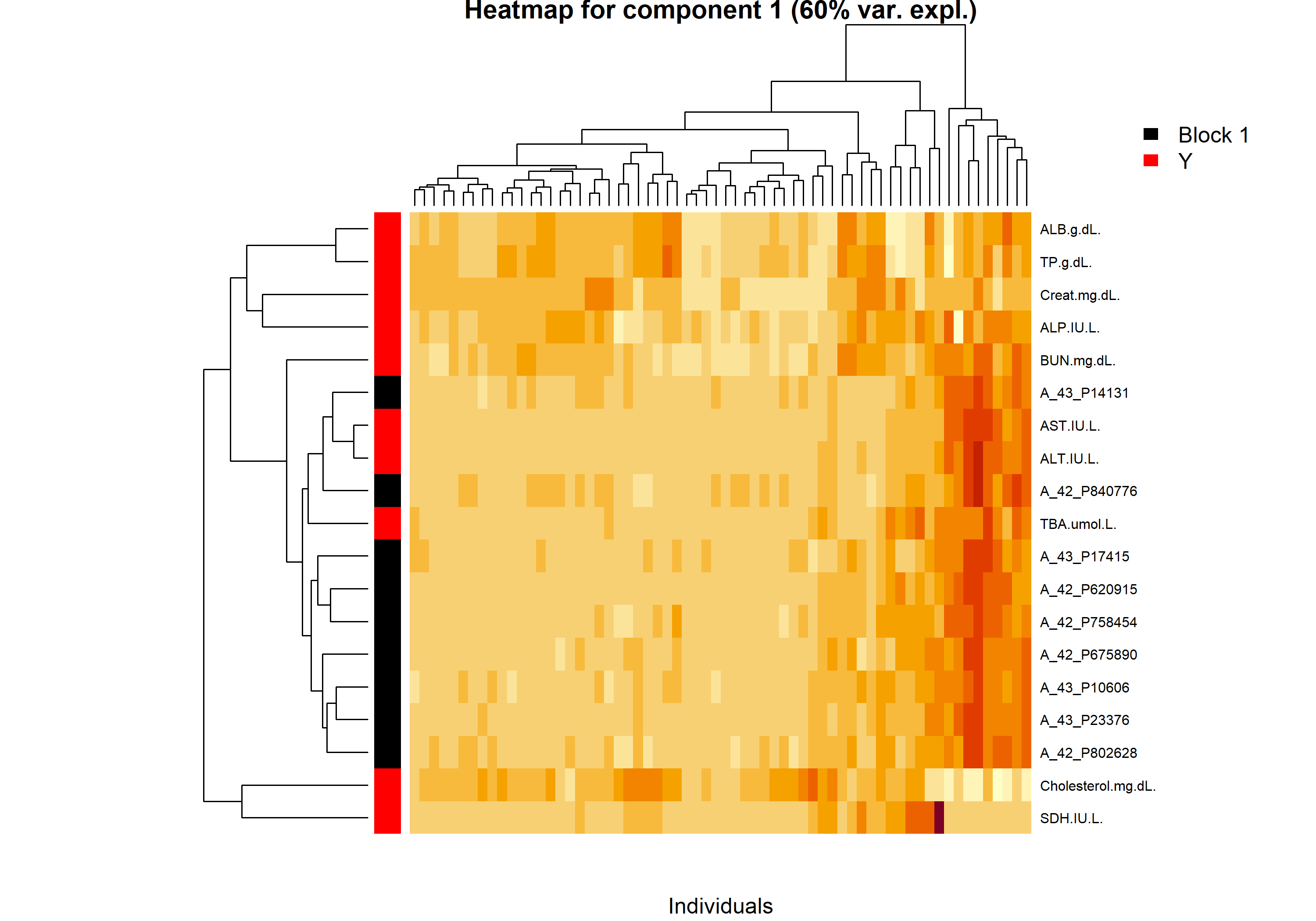Each variable has been normalized separately. Dendrogram are used in the individual and in the variable directions using hclust similarities. #### Summary Method A summary method explains more precisely the model. summary(mddsPLS_model_reg)  ## =============================================================== ## ddsPLS object description ## =============================================================== ## ## Number of blocks: 1 ## Number of dimensions: 3 ## Regularization coefficient: 10 ## Number of individuals: 64 ## Number of variables in Y part: 10 ## Model built in mode regression ## Koh-Lanta process realized in 0 iterations ## ## ## Variance Explained (%) ## ------------------------------------------- ## Total Y variance explained by all the Super Components ##  66 ## Total Y variance explained by each Super Component ## Super Comp. 1 Super Comp. 2 Super Comp. 3 ## 60 55 54 ## ## Marginal Y variable variance explained by each Super Component ## Super Comp. 1 Super Comp. 2 Super Comp. 3 ## BUN.mg.dL. 69.0 61.0 54.0 ## Creat.mg.dL. 15.0 19.0 9.0 ## TP.g.dL. 1.7 4.6 15.0 ## ALB.g.dL. 15.0 4.6 7.6 ## ALT.IU.L. 96.0 86.0 84.0 ## SDH.IU.L. 10.0 18.0 25.0 ## AST.IU.L. 95.0 84.0 83.0 ## ALP.IU.L. 37.0 36.0 33.0 ## TBA.umol.L. 84.0 82.0 90.0 ## Cholesterol.mg.dL. 66.0 57.0 46.0 ## ## Total Y variance explained by each component of each block ## Comp. 1 Comp. 2 Comp. 3 ## Block 1 60 54 55 ## ## ## RV coefficient ## ------------------------------------------------------- ## Total Y with all the Super Components ##  0.32 ## ## Total Y with each Super Component ## Super Comp. 1 Super Comp. 2 Super Comp. 3 ## 0.36 0.30 0.29 ## ## Each Y variable with each Super Component ## Super Comp. 1 Super Comp. 2 Super Comp. 3 ## BUN.mg.dL. 0.47000 0.3800 0.2900 ## Creat.mg.dL. 0.02300 0.0350 0.0082 ## TP.g.dL. 0.00028 0.0021 0.0220 ## ALB.g.dL. 0.02100 0.0021 0.0058 ## ALT.IU.L. 0.92000 0.7500 0.7100 ## SDH.IU.L. 0.01000 0.0310 0.0600 ## AST.IU.L. 0.91000 0.7000 0.6900 ## ALP.IU.L. 0.14000 0.1300 0.1100 ## TBA.umol.L. 0.70000 0.6700 0.8100 ## Cholesterol.mg.dL. 0.43000 0.3300 0.2100 ## ## Total Y with each component of each block ## Comp. 1 Comp. 2 Comp. 3 ## Block 1 0.36 0.29 0.3 ## ## ## Missing value information ## ------------------------------------------- ## ## X1 Total ## Number of variables 3116 3116 ## Number of missing samples 0 0 ## Proportion of missing samples (%) 0 0 ## ## ## mddsPLS results ## ------------------------------------------- ## ## At most 10 variable(s) can be selected in the X part ## ## ## ---- For each block of X, are selected ## Super Comp. 1 Super Comp. 2 Super Comp. 3 ## 1 9 9 1 ## ---- For the Y block, are selected ## @ (2,2,1) variable(s) ## ## ## --------------------------------------------------------------- ## ===============================================================  #### Get selected variables Output var_selected permits to get the selected variables per block. This is a list filled with \code{2R}-column matrices corresponding to the non nul coefficients of each block on the \emph{Super-Components}. They are ordered according to their absolute value on the first Super-Component, in a decreasing way. mddsPLS_model_reg$var_selected[]

##              Weights_comp_1 Weights_comp_2 Weights_comp_3
## A_42_P620915   -0.591212926              0    -0.45490352
## A_42_P809565    0.000000000              1     0.00000000
## A_42_P840776   -0.156508320              0    -0.61046161
## A_43_P14131    -0.737716168              0     0.37689499
## A_43_P23376    -0.069399953              0    -0.21628484
## A_42_P675890   -0.031981983              0    -0.16317508
## A_43_P10606    -0.029507817              0    -0.15055165
## A_43_P17415    -0.273185553              0     0.41434680
## A_42_P758454   -0.019127771              0    -0.09759168
## A_42_P802628   -0.006414699              0    -0.03272840
##              Super_Weights_comp_1 Super_Weights_comp_2
## A_42_P620915          0.591212926           0.45490352
## A_42_P809565          0.000000000           0.00000000
## A_42_P840776          0.156508320           0.61046161
## A_43_P14131           0.737716168          -0.37689499
## A_43_P23376           0.069399953           0.21628484
## A_42_P675890          0.031981983           0.16317508
## A_43_P10606           0.029507817           0.15055165
## A_43_P17415           0.273185553          -0.41434680
## A_42_P758454          0.019127771           0.09759168
## A_42_P802628          0.006414699           0.03272840
##              Super_Weights_comp_3
## A_42_P620915                    0
## A_42_P809565                   -1
## A_42_P840776                    0
## A_43_P14131                     0
## A_43_P23376                     0
## A_42_P675890                    0
## A_43_P10606                     0
## A_43_P17415                     0
## A_42_P758454                    0
## A_42_P802628                    0


### Cross Validation {.tabset}

The cross-validation process is started in a leave-one-out design along $$1$$ dimension. NCORES fixes the number of cores in the paralellized process, n_lambda fixes the number of regularization coefficients to be tested.

n_lambda <- 50
NCORES <- 7

res_cv_reg_L0 <- perf_mddsPLS(Xs = X,Y = Y,
R = 1,L0s=1:50,
mode = "reg",NCORES = NCORES,kfolds = "loo")

res_cv_reg <- perf_mddsPLS(Xs = X,Y = Y,
R = 1,lambda_min=0.5,n_lambda=n_lambda,
mode = "reg",NCORES = NCORES,kfolds = "loo")


#### Error Plot {.tabset}

A plot method plots the results. plot_mean permits to add the mean of the Y variable prediction errors. legend_names permits to add a legend with the names of the Y variables. pos_legend permits to choose the position of the legend. which_sd_plot picks the Y variables whose standard error must be drawn.

##### Using $$L_0$$ paradigm
plot(res_cv_reg_L0,which_sd_plot = c(5,7),ylim=c(0,1.1),alpha.f = 0.4,
plot_mean = T,legend_names = colnames(Y),pos_legend = "bottomright",no_occurence = T)

## $optim_para_all ##  50 ## ##$optim_para_one
##  18
##
## attr(,"class")
##  "plot.perf_mddsPLS"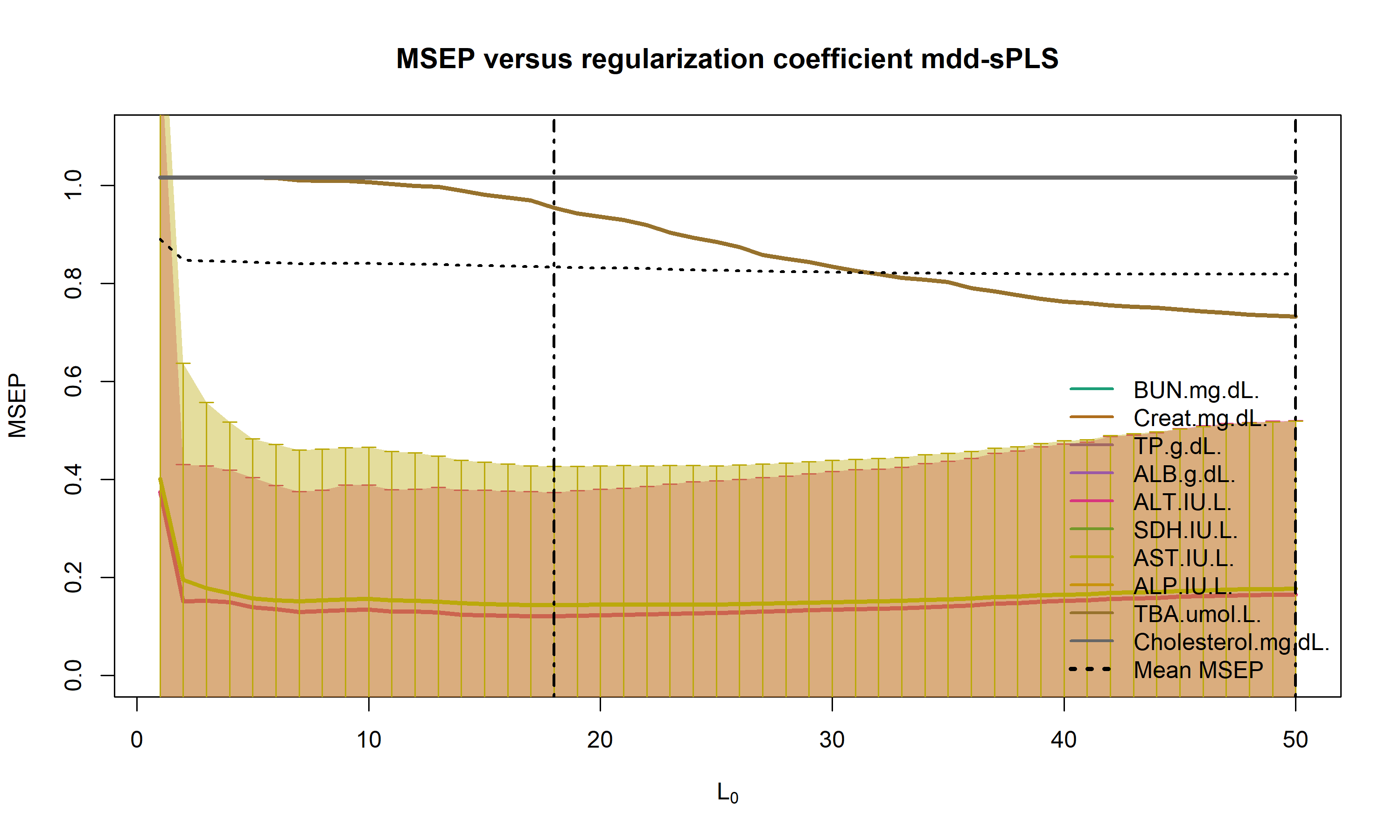Minimum for $$L_0\approx 18$$.

##### Using $$\lambda$$ paradigm
plot(res_cv_reg,which_sd_plot = c(5,7),ylim=c(0,1.1),
alpha.f = 0.4,plot_mean = T,legend_names = colnames(Y),
no_occurence = T)

## $optim_para_all ##  0.5 ## ##$optim_para_one
##  0.8456114
##
## attr(,"class")
##  "plot.perf_mddsPLS"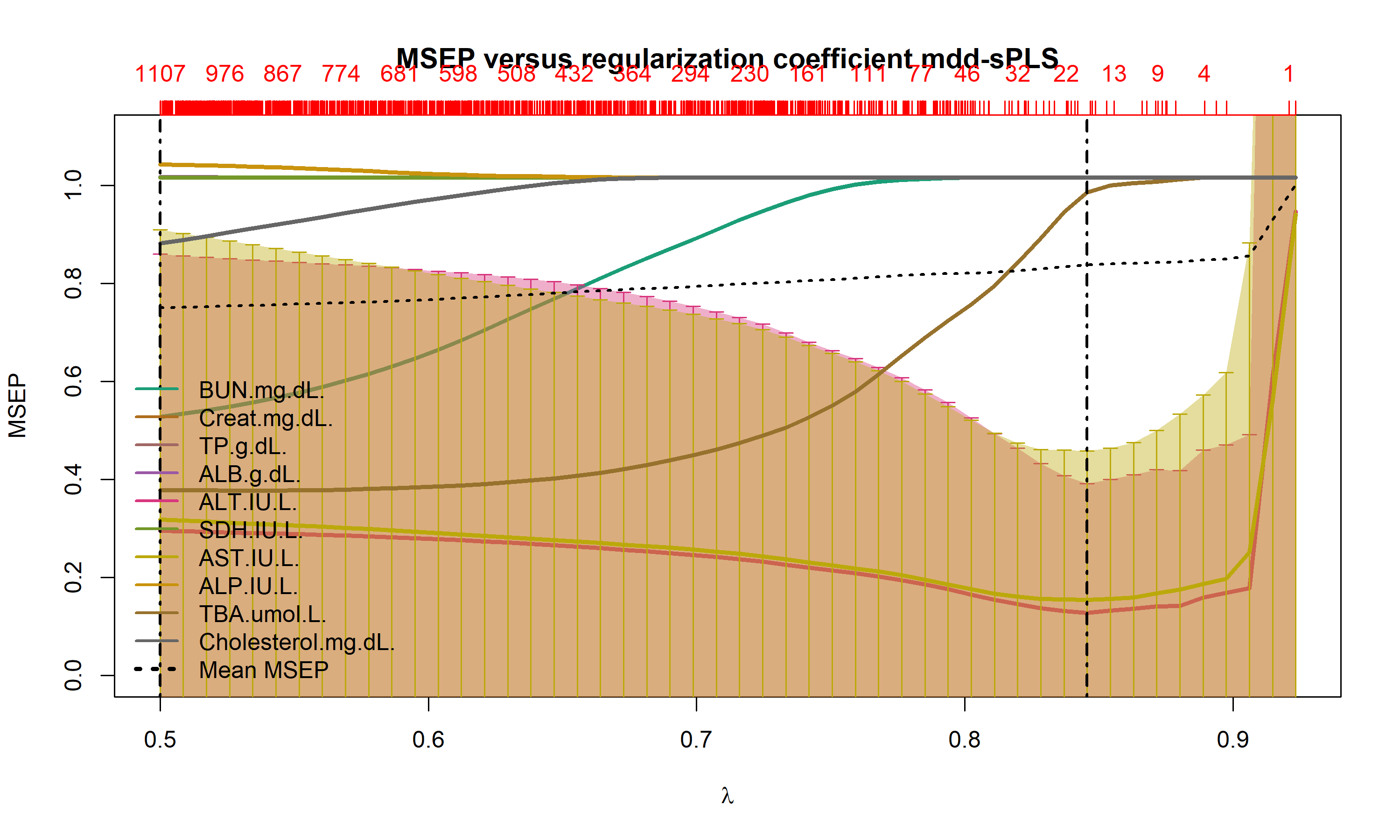Minimum for $$\lambda\approx0.85$$.

##### Observations

Are also plotted vertical lines representing:

• The global minimum,
• The minimum of the Mean MSEP.

The two panels building this plot are headed with numbers. Red for the error curves and blue for the $$\mathbf{Y}$$ variable occurence curves. It represents the number of variables selected in the $$\mathbf{X}$$ and in the $$\mathbf{Y}$$ respectively for the model built on the matrices given to the function.

If one would not prefer to see the occurence plot. The parameter no_occurence, when set to TRUE, permits to hide it.

#### Summary Method

A summary methods explains more precisely the model. The parameter plot_res_cv uses the plot method. Are also presented the statistical results of the algorithm (convergence for each fold, time of computation).

summary(res_cv_reg,plot_res_cv =F)

## ======================================================
##       Cross-Validation ddsPLS object description
## ======================================================
##
## Number of blocks: 1
## Number of individuals: 64
## Number of variables in Y part: 10
## Model built in mode regression
##
##
##     Missing value information
## ---------------------------------
##
##                                     X1 Total
## Number of variables               3116  3116
## Number of missing samples            0     0
## Proportion of missing samples (%)    0     0
##
##
##      Cross-Validation results
## ---------------------------------
##
##    R    lambda Nb.of.convergences.VS.nb.of.fold
## 1  1 0.5000000                             0/64
## 2  1 0.5086403                             0/64
## 3  1 0.5172806                             0/64
## 4  1 0.5259209                             0/64
## 5  1 0.5345611                             0/64
## 6  1 0.5432014                             0/64
## 7  1 0.5518417                             0/64
## 8  1 0.5604820                             0/64
## 9  1 0.5691223                             0/64
## 10 1 0.5777626                             0/64
## 11 1 0.5864029                             0/64
## 12 1 0.5950431                             0/64
## 13 1 0.6036834                             0/64
## 14 1 0.6123237                             0/64
## 15 1 0.6209640                             0/64
## 16 1 0.6296043                             0/64
## 17 1 0.6382446                             0/64
## 18 1 0.6468849                             0/64
## 19 1 0.6555251                             0/64
## 20 1 0.6641654                             0/64
## 21 1 0.6728057                             0/64
## 22 1 0.6814460                             0/64
## 23 1 0.6900863                             0/64
## 24 1 0.6987266                             0/64
## 25 1 0.7073669                             0/64
## 26 1 0.7160071                             0/64
## 27 1 0.7246474                             0/64
## 28 1 0.7332877                             0/64
## 29 1 0.7419280                             0/64
## 30 1 0.7505683                             0/64
## 31 1 0.7592086                             0/64
## 32 1 0.7678489                             0/64
## 33 1 0.7764891                             0/64
## 34 1 0.7851294                             0/64
## 35 1 0.7937697                             0/64
## 36 1 0.8024100                             0/64
## 37 1 0.8110503                             0/64
## 38 1 0.8196906                             0/64
## 39 1 0.8283309                             0/64
## 40 1 0.8369711                             0/64
## 41 1 0.8456114                             0/64
## 42 1 0.8542517                             0/64
## 43 1 0.8628920                             0/64
## 44 1 0.8715323                             0/64
## 45 1 0.8801726                             0/64
## 46 1 0.8888129                             0/64
## 47 1 0.8974531                             0/64
## 48 1 0.9060934                             0/64
## 49 1 0.9147337                             0/64
## 50 1 0.9233740                             0/64
##    Mean.AND.sd.time.of.computation
## 1                      0.29(0.025)
## 2                      0.29(0.029)
## 3                      0.29(0.029)
## 4                      0.29(0.031)
## 5                      0.29(0.027)
## 6                      0.29(0.027)
## 7                      0.29(0.024)
## 8                      0.29(0.022)
## 9                      0.29(0.021)
## 10                     0.29(0.022)
## 11                     0.29(0.023)
## 12                      0.29(0.03)
## 13                     0.29(0.027)
## 14                     0.29(0.026)
## 15                     0.29(0.024)
## 16                      0.3(0.024)
## 17                     0.29(0.028)
## 18                      0.3(0.028)
## 19                     0.29(0.028)
## 20                     0.29(0.027)
## 21                     0.29(0.023)
## 22                     0.29(0.025)
## 23                      0.3(0.025)
## 24                     0.29(0.023)
## 25                     0.29(0.022)
## 26                     0.29(0.029)
## 27                     0.29(0.021)
## 28                     0.29(0.022)
## 29                      0.29(0.02)
## 30                     0.29(0.019)
## 31                     0.29(0.024)
## 32                     0.29(0.025)
## 33                     0.29(0.027)
## 34                     0.29(0.021)
## 35                     0.29(0.024)
## 36                     0.29(0.025)
## 37                     0.29(0.023)
## 38                     0.29(0.019)
## 39                     0.29(0.027)
## 40                     0.29(0.026)
## 41                     0.29(0.023)
## 42                     0.29(0.026)
## 43                     0.29(0.021)
## 44                     0.29(0.028)
## 45                     0.29(0.023)
## 46                     0.29(0.023)
## 47                     0.29(0.029)
## 48                     0.29(0.024)
## 49                     0.29(0.023)
## 50                     0.29(0.023)
##
##
## ------------------------------------------------------
## ======================================================


The Cross-Validation results is a matrix with 4 columns and N rows, each row corresponds to a couple $$(R,\lambda)$$ teste. Each column correpsonds to:

• R: The number of components built,
• lambda: The regularization coefficient,
• Nb.of.convergences.VS.nb.of.fold: The number of models, in the cross-validation process, which have converged against the total number of models built.
• Mean.sd..time.of.computation: The mean time of computation for bulding a model and, in parenthesis, its standard deviation.

# Multi-block

Two different analyses are detailed here, the first one presents the tool and the second one permits to simulate a data-set and details its analysis thanks to the ddsPLS tool.

## Toy example {.tabset}

In the case of missing values it is possible to visualize the missing positions. Let us just consider a 3-blocks toy-example case, based on the previous dataset, such as

data("liverToxicity")
X <- scale(liverToxicity$gene) Y <- scale(liverToxicity$clinic)
p1=p2 <- 1000
p3 <- ncol(X)-p1-p2
Xs <- list(Block1=X[,1:p1],Matrix2=X[,p1+1:p2],Other_Variables=X[,p1+p2+1:p3])
Xs$Block1[1:10,] <- NA Xs$Matrix2[5+1:10,] <- NA
Xs$Other_Variables[10+1:20,] <- NA model_multi_vizu <- mddsPLS(Xs,Y,lambda = 0.8,R = 3)  ### Plot Method {.tabset} The Plot mehtod permits many types of representation. Among which • weights which shows all the weights under barplot representations. If super is set to TRUE, then the super-weights are represented. In the mono-block case, super-weights are equal to weights. • heatmap which shows the selected variables (for $$\mathbf{X}$$ and $$\mathbf{Y}$$) for the all individuals. The following sections detailed the discussed method though those parametizations. #### Scaled Super-Weight representation {.tabset} The following figure permits to represent the scaled super-weights for blocks 1, 2 and block Y. mar_left parameter adds extra values on the left of the plot, usefull to see variable names. Only the first cuper-component is plotted. plot(model_multi_vizu,vizu = "weights",super = T,comp=1 ,addY = T, mar_left = 5,mar_bottom = 3,reorder_Y = T)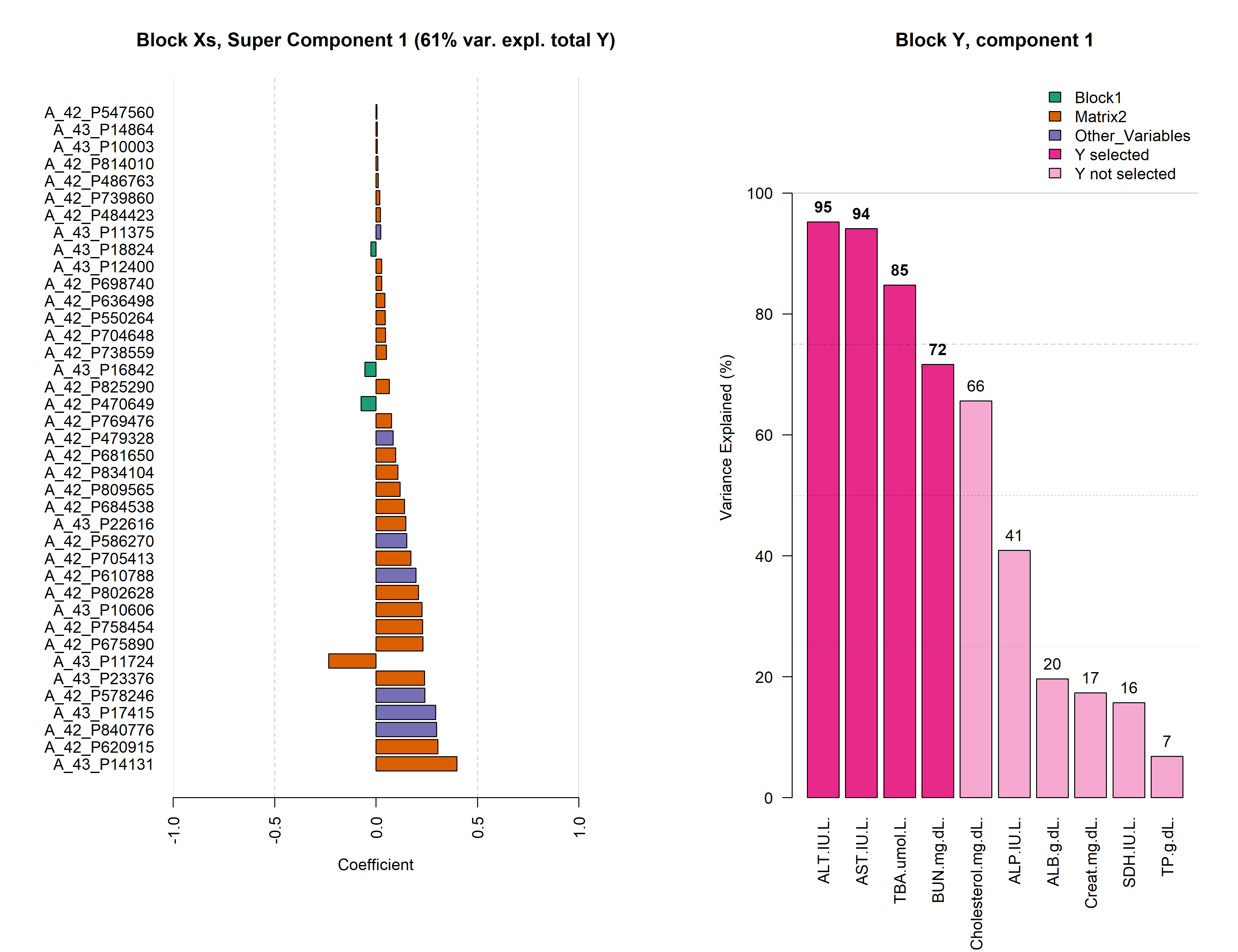#### Heatmap representation {.tabset} Heatmap permit to appreciate the discriminative aspect of variables according to their individual representations. It has been decided to plot heatmaps component per component, only the first component in the case of that example. Each variable has been normalized separately. Dendrogram are used in the individual and in the variable directions using hclust similarities. plot(model_multi_vizu,vizu = "heatmap",comp = 1)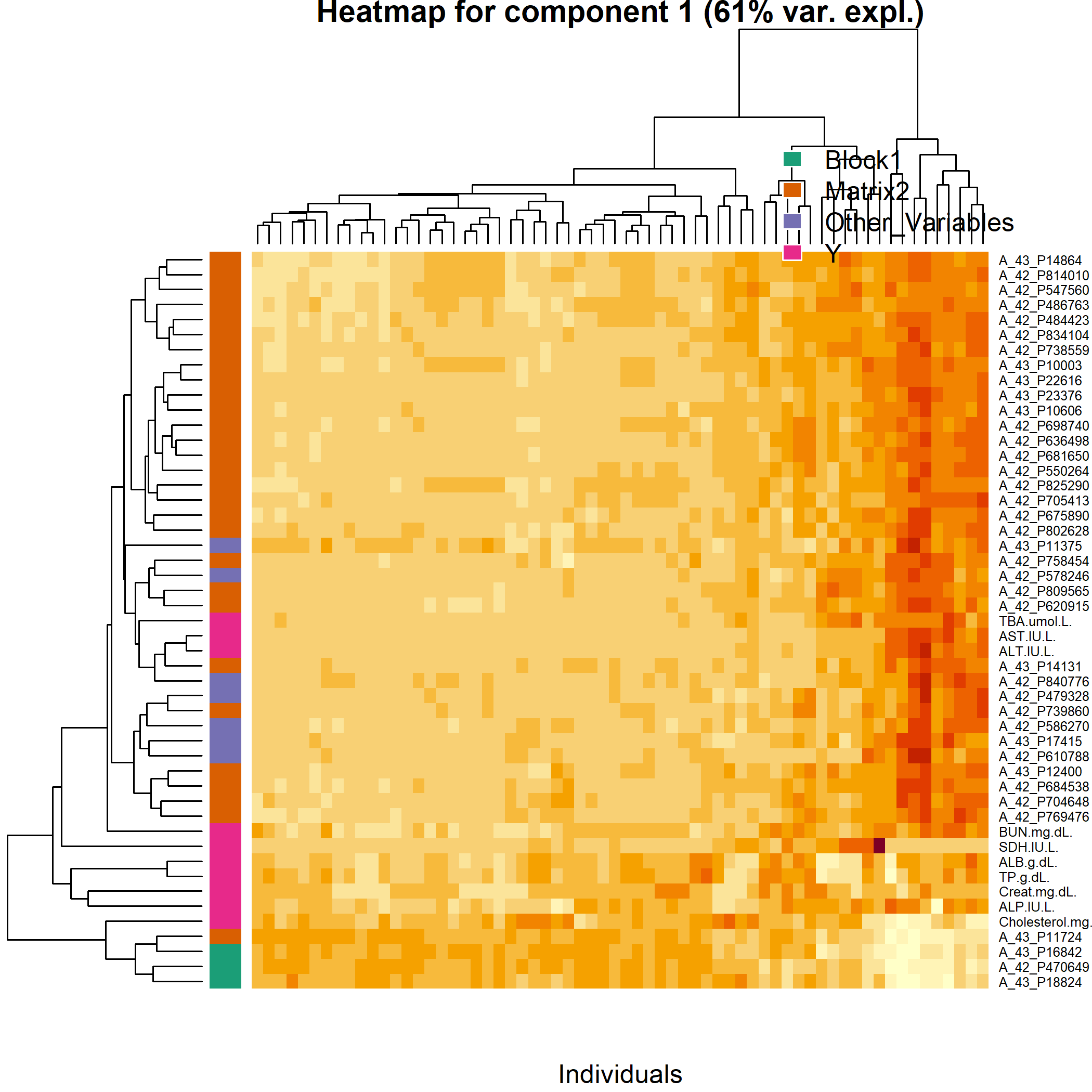### Summary Method A summary method explains more precisely the model. summary(model_multi_vizu)  ## =============================================================== ## ddsPLS object description ## =============================================================== ## ## Number of blocks: 3 ## Number of dimensions: 3 ## Regularization coefficient: 0.8 ## Number of individuals: 64 ## Number of variables in Y part: 10 ## Model built in mode regression ## Koh-Lanta process realized in 2 iterations ## ## ## Variance Explained (%) ## ------------------------------------------- ## Total Y variance explained by all the Super Components ##  71 ## Total Y variance explained by each Super Component ## Super Comp. 1 Super Comp. 2 Super Comp. 3 ## 61 55 47 ## ## Marginal Y variable variance explained by each Super Component ## Super Comp. 1 Super Comp. 2 Super Comp. 3 ## BUN.mg.dL. 72.0 70 44.0 ## Creat.mg.dL. 17.0 23 8.7 ## TP.g.dL. 6.8 20 30.0 ## ALB.g.dL. 20.0 32 26.0 ## ALT.IU.L. 95.0 82 65.0 ## SDH.IU.L. 16.0 15 39.0 ## AST.IU.L. 94.0 78 64.0 ## ALP.IU.L. 41.0 42 32.0 ## TBA.umol.L. 85.0 70 84.0 ## Cholesterol.mg.dL. 66.0 60 28.0 ## ## Total Y variance explained by each component of each block ## Comp. 1 Comp. 2 Comp. 3 ## Block1 56 46 43 ## Matrix2 60 49 56 ## Other_Variables 60 31 51 ## ## ## RV coefficient ## ------------------------------------------------------- ## Total Y with all the Super Components ##  0.3 ## ## Total Y with each Super Component ## Super Comp. 1 Super Comp. 2 Super Comp. 3 ## 0.37 0.30 0.22 ## ## Each Y variable with each Super Component ## Super Comp. 1 Super Comp. 2 Super Comp. 3 ## BUN.mg.dL. 0.5100 0.490 0.1900 ## Creat.mg.dL. 0.0300 0.051 0.0076 ## TP.g.dL. 0.0047 0.039 0.0890 ## ALB.g.dL. 0.0390 0.100 0.0690 ## ALT.IU.L. 0.9100 0.680 0.4200 ## SDH.IU.L. 0.0250 0.023 0.1500 ## AST.IU.L. 0.8900 0.610 0.4000 ## ALP.IU.L. 0.1700 0.180 0.1000 ## TBA.umol.L. 0.7200 0.490 0.7100 ## Cholesterol.mg.dL. 0.4300 0.360 0.0770 ## ## Total Y with each component of each block ## Comp. 1 Comp. 2 Comp. 3 ## Block1 0.32 0.210 0.19 ## Matrix2 0.37 0.240 0.31 ## Other_Variables 0.37 0.095 0.26 ## ## ## Missing value information ## ------------------------------------------- ## ## Block1 Matrix2 Other_Variables Total ## Number of variables 1000.0 1000.0 1116.0 3116.0 ## Number of missing samples 10.0 10.0 20.0 40.0 ## Proportion of missing samples (%) 15.6 15.6 31.2 20.8 ## ## ## mddsPLS results ## ------------------------------------------- ## ## At most 39 variable(s) can be selected in the X part ## ## ## ---- For each block of X, are selected ## Super Comp. 1 Super Comp. 2 Super Comp. 3 ## Block1 3 3 3 ## Matrix2 29 29 29 ## Other_Variables 7 7 7 ## ---- For the Y block, are selected ## @ (4,4,4) variable(s) ## ## ## --------------------------------------------------------------- ## ===============================================================  ### Get selected variables Output var_selected permits to get the selected varaibles per block. This is a list filled with \code{2R}-column matrices corresponding to the non nul coefficients of each block on the \emph{Super-Components}. They are ordered according to their absolute value on the first Super-Component, in a decreasing way. Only $$2$$ significance digits are kept here for convenience. Here is represented its value for the first block model_multi_vizu$var_selected[]

##              Weights_comp_1 Weights_comp_2 Weights_comp_3
## A_43_P16842       0.8112084     -0.5844501     0.01894704
## A_43_P18824       0.2021202      0.3106499     0.92878632
## A_42_P470649      0.5487152      0.7496097    -0.37013102
##              Super_Weights_comp_1 Super_Weights_comp_2
## A_43_P16842           -0.05547870          -0.03013106
## A_43_P18824           -0.02591159          -0.21819422
## A_42_P470649          -0.07391688           0.02654694
##              Super_Weights_comp_3
## A_43_P16842           -0.25492936
## A_43_P18824            0.01562498
## A_42_P470649           0.03112857